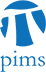## Discrete Math Seminar: Estimating the entropy of Z^2 shifts of finite type

• Date: 10/27/2009
Lecturer(s):
Ronnie Pavlov (UBC)
Location:

University of British Columbia

Description:

In symbolic dynamics, a Z^d shift of finite type (or SFT) is the set of all ways to assign elements from a finite alphabet A to all sites of Z^d, subject to local rules about which elements of A are allowed to appear next to each other. A fundamental number associated to any SFT is its (topological) entropy, which is, roughly speaking, the exponential growth rate of the number of allowed patterns of size n.

The entropy of any Z SFT is easily computable (it is the log of an algebraic number). However, for d > 1, the situation becomes more complex. There are in fact only a few nontrivial examples of Z^2 SFTs whose entropies have explicit closed forms.

It is natural then to try to at least estimate these entropies. We will discuss some of the difficulties involved in doing this, and present a way of approximating entropy for a class of Z^2 SFTs by way of some easier-to-compute entropies of associated Z SFTs.

As a corollary of this technique, we can show that the entropy of any
Z^2 SFT in this class is computable in polynomial time.

Schedule:

16:00 - 17:00, WMAX 216.